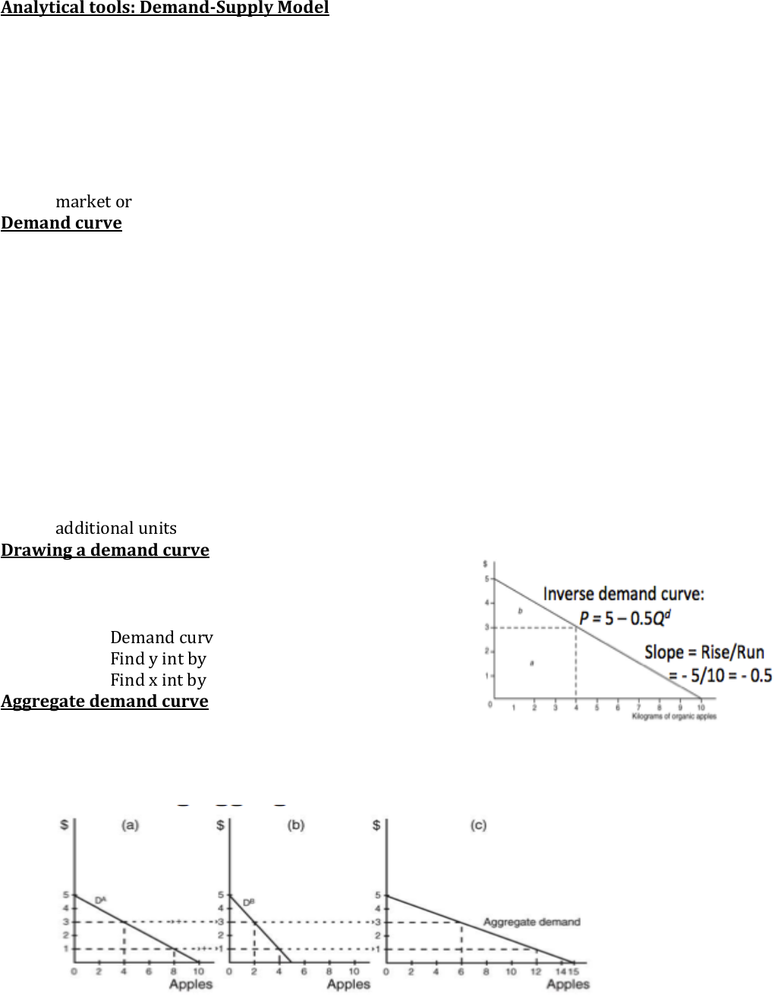Textbook Notes (280,000)
CA (160,000)
UofL (100)
Chapter 3

# Economics 3220 Chapter Notes - Chapter 3: Aggregate Demand, Aggregate Supply, Demand Curve

Department
Economics
Course Code
Economics 3220
Professor
Ali Kamar
Chapter
3

Page:
of 4Chapter 3
Analytical tools: Demand-Supply Model
Basic analytical tool in environmental economics starts with standard
competitive market model
o It helps us understand what happens when competitive market fails
to account for pollution externality and how government actions can
help
o It also helps us quantify the benefits and costs with and without such
government actions/policies
Competitive market model uses market or aggregate demand curve and
market or aggregate supply curve
Demand curve
The demand curve is a relationship between quantity demanded and market
price
The price reflects what an individual is willing to pay (WTP) for consuming
each unit of the good
Usually, individuals are willing to pay high price for the first few units but
lower price for additional units- marginal WTP falls
Because with limited budget, opportunity cost of more units increases. So,
individuals are not willing to pay as much for additional units as for the first
unit.
This falling mWTP give the downward sloping demand curve. So, the demand
curve is also known as the marginal willingness to pay (mWTP) curve
It is also known as the marginal benefit (MB) curve as each point on the
demand curve reflects the monetary value of benefits of consumption from
Drawing a demand curve
Always written as function of Q. Drawn as function
of P.
Example:
o Demand curve Q=10-2p. inverse is P=5-0.5Q
o Find y int by setting Q=0. P=5
o Find x int by setting P=0. Q=10.
Aggregate demand curve
Aggregate demand curve is derived as the horizontal
summation of two or more individuals’ demand curves at each price
Aggregate demand curve is also drawn in inverse
Kink occurs if vertical intercepts are different
Consumer surplus
Individuals derive benefits from consumption
The dollar value of the benefits of consumption is the difference between
what individuals are willing to pay (tWTP) and what hey actually pay (TE)
This is also known as consumer surplus (CS) the excess beenfits received
from consuming a good over the amount pad for it
CS= tWTP/ TE
o tWTP= area under the demand (or mWTP) curve up to the quantity
purchased
o TE= area (P X Q)
CS= are under the demand curve and above the price line up to the quantity
purchased.
Supply curve
supply curve is the relationship between quantity supplied (Q)and its
marginal cost (MC)
Firms use inputs such as raw materials, labour and machinery to produce
output
Once used to produce a good these inputs are not available for other sues-
this is their opportunity cost of production
To produce additional units of a good, more inputs are needed
opportunity cost rises
The rising OC defines firm’s marginal cost curve or supply curve
Profit maximizing producers set MC=P this is why supply curve is also a
relation between Q and P
Drawing a supply curve
Written as function of Q.
Drawn as function of P.
Example
o Q= 4+3P
Inverse P= 4/3 +
1/3 Q
o X int
P=0. Q= -4
o Y int
Q=0. P=4/3
Aggregate supply curve
Aggregate supply curve is derived by the horizontal summation of two or
more firm’s supply serves at each price
Aggregate supply curve is drawn in the inverse
Aggregate supply curve has a kink if the vertical intercepts are not the same